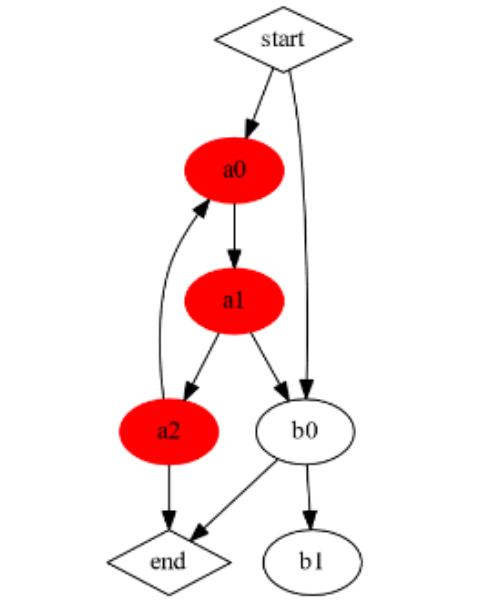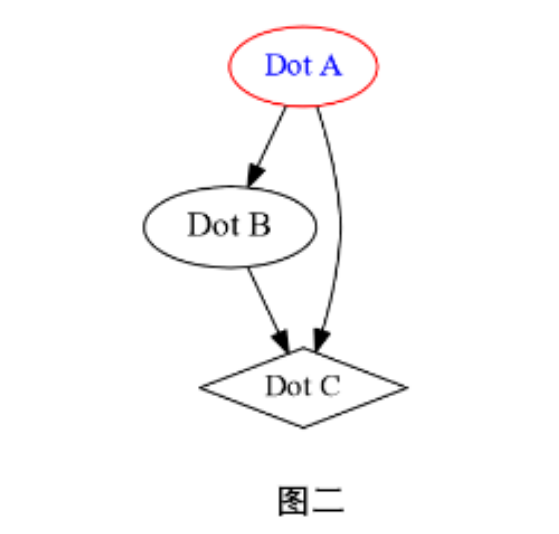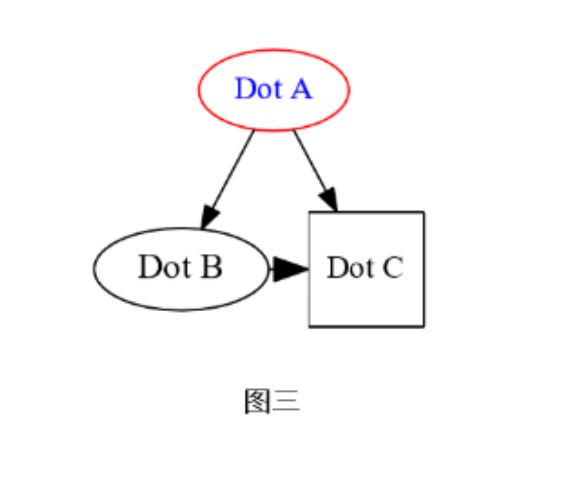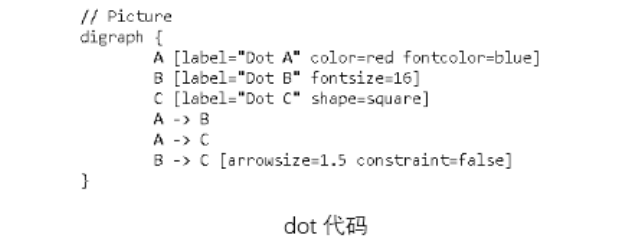2023
05-03

# python graphviz 教程（Python图形可视化之graphviz）graphviz库通过使用图(Digraph/graph)、节点(node)和边(edge)等来绘制关系图或流程图，相应地不同结构有着不同属性，使用时可通过添加或删除属性来改变图形显示样式。接下来，我们先通过简单图的绘制来了解不同属性吧~

from graphviz import Digraphdot=Digraph(comment='Picture')然后，添加节点，并设置相应属性。在下面的程序中，我们设置节点A的标签为'Dot A'，文本颜色（fontcolor）为蓝色，节点框（color）的颜色为红色；设置节点B的标签为'Dot B'，文字大小（fontsize）为16；同理，设置节点C的形状（shape）为菱形：
dot.node('A','Dot A',fontcolor='blue',color='red')dot.node('B','Dot B',fontsize='16')dot.node('C','Dot C',shape='diamond')最后，设置节点方向及边属性，并查看图形：
dot.edges(['AB','AC'])

dot.edge('B','C')

dot.view()from graphviz import Digraph

dot=Digraph(comment='Picture')

dot.node('A','Dot A',fontcolor='blue',color='red')

dot.node('B','Dot B',fontsize='16')

dot.node('C','Dot C',shape='square')   #设置节点C的形状为方形

dot.edges(['AB','AC'])dot.edge('B','C',arrowsize='1.5',constraint='false')   #设置BC节点之间的有向标的箭头大小为1.5，并添加constraint属性设置是否根据边来影响节点的排序

dot.view()print(dot.source)

dot.render('D:\picture',view=True)from graphviz import Digraph

grap_g = Digraph("G",format="pdf")

sub_g0=Digraph(comment="process1",node_attr={"style":"filled","color":"red"})

#一般可在单个节点处设置其属性，也可在创建图表时通过添加"node_attr"属性来设置节点样式：在这里，设置节点颜色为红色并进行填充

sub_g0.node("a0","a0")

sub_g0.node("a1","a1")

sub_g0.node("a2","a2")

sub_g0.edge("a0","a1")

sub_g0.edge("a1","a2")

sub_g0.edge("a2", "a0")

sub_g1=Digraph(comment="process1",graph_attr={"style":'filled'})

sub_g1.node("B","b0")

sub_g1.node("C","b1")

sub_g1.edges(["BC"])

grap_g.node("start", label="start",shape="diamond")

grap_g.node("end", label="end", shape="diamond")

grap_g.subgraph(sub_g0)

grap_g.subgraph(sub_g1)

grap_g.edge("start","a0")

grap_g.edge("start","B")

grap_g.edge("a1","B")

grap_g.edge("a2","end")

grap_g.edge("B","end")

grap_g.view()